### Home > CALC > Chapter 12 > Lesson 12.2.2 > Problem12-83

12-83.
1. Multiple Choice: A particle moves in the plane according to the equations x(t) = cos πt and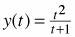. What is the magnitude of the velocity vector at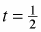? Homework Help ✎

1.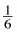2.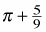3.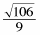4.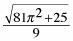5. 0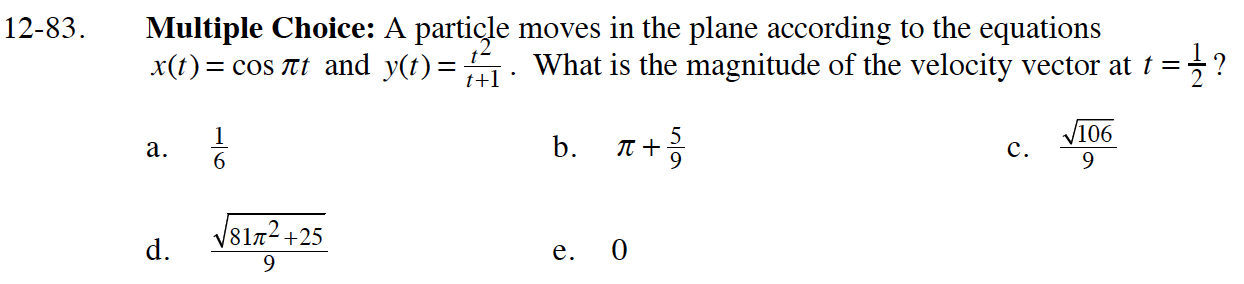$\vec{v}(0.5)=\langle -\pi\sin(\pi/2),\frac{0.5^2+1}{(1.5)^2}\rangle=\langle -\pi,\frac{5}{9}\rangle$

Sketch this vector, then use the Pythagorean Theorem to determine its magnitude.